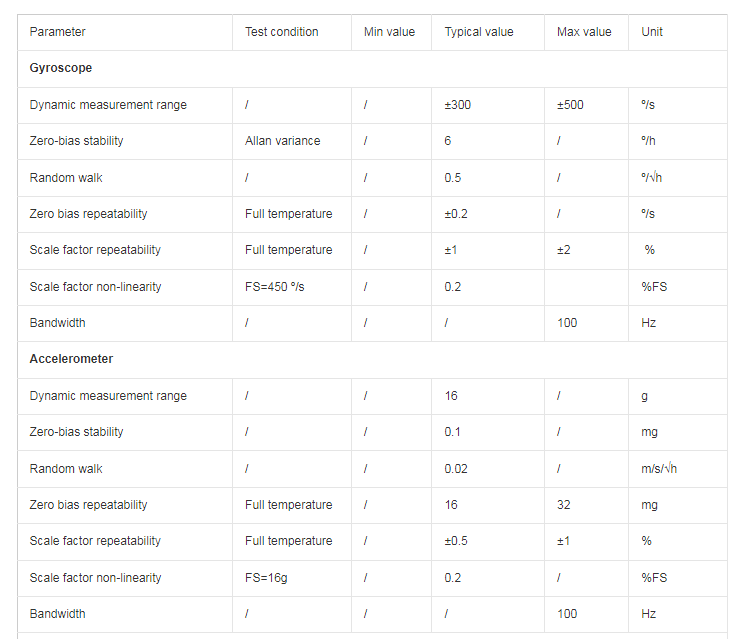Application

# What is an IMU?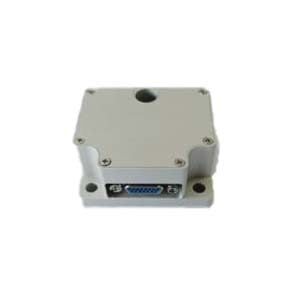The full name of IMU is inertial measurement unit, which generally refers to a 6-axis sensor, consisting of three single-axis accelerometers and three single-axis gyroscopes. The 3-axis represents the three coordinate axes under the XYZ plane. The 3-axis gyroscope measures the angular velocity on each axis, and the accuracy is generally °/s, which is the number of degrees it can travel per second when rotating according to this trend. The 3-axis accelerometer measures the gravity acceleration experienced by each axis. For example, when the sensor is placed horizontally on a level ground, it is theoretically only subject to the negative gravity acceleration along the z-axis, which is 9.8 m/s^2. The accelerometer detects the acceleration signals of the object in three independent axes of the carrier coordinate system, and the gyroscope detects the angular velocity signal of the carrier relative to the navigation coordinate system. After processing these signals, the attitude of the object can be obtained. calculated.

Inertial Measurement Unit Definition

In a narrow sense, IMU is equipped with gyroscopes and accelerometers on three orthogonal axes, with a total of 6 degrees of freedom, to measure the angular velocity and acceleration of objects in three-dimensional space, which is what we call “IMU”. 6-axis IMU”;

There is an easy-to-understand box model of the accelerometer on the web. We assume that there is a ball placed in a square box of the same size in a weightless environment, as shown in the figure.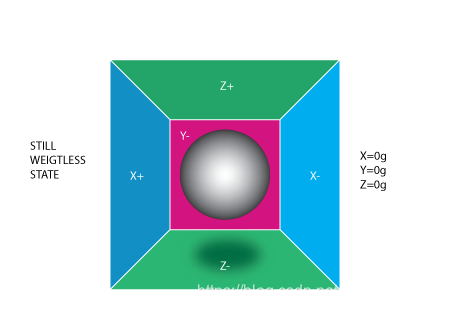At this time, if there is an acceleration to the left, as shown in the figure.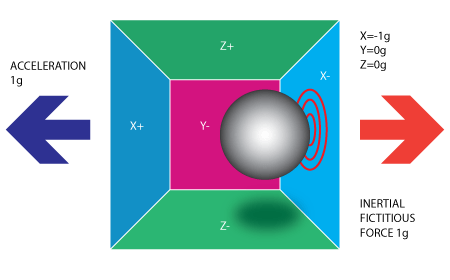Then a force can be detected on the right side of the box. If you are on the earth, there is a gravitational acceleration as shown in the figure.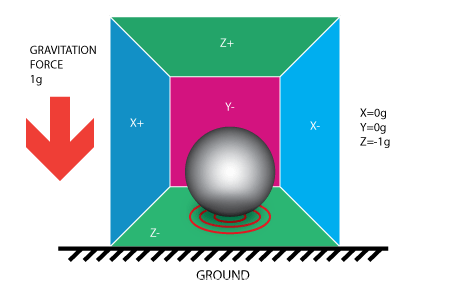At this time, we can calculate the attitude of the object based on the components of the acceleration of gravity in each direction, but the horizontal yaw angle cannot be determined perpendicular to the acceleration of gravity. Here we also only need to know the following two points. The accelerometer can measure three axes. The acceleration accelerometer cannot calculate the yaw angle in the horizontal direction.

The accelerometer detects the acceleration signal of the object on three independent axes of the carrier coordinate system, the gyroscope detects the angular velocity signal of the carrier relative to the navigation coordinate system, and measures the angular velocity and acceleration of the object in the carrier coordinate system. three-dimensional space and calculate the pose of the object. The core value of IMU in navigation is irreplaceable. In order to improve its reliability, each single axis can be equipped with a wider variety of sensors. In order to ensure measurement accuracy, generally the IMU should be installed at the center of gravity of the object being measured.

Broadly speaking, an IMU can add a magnetometer to an accelerometer and a gyroscope to form what is now known as a “9-axis IMU”.

Magnetometer Simply put, a magnetometer is a compass, which just makes up for the problem of the horizontal yaw angle that the accelerometer cannot measure. It is worth noting that when calculating the attitude with the magnetometer, the formula anglez=−arctan2(mny,mnx) cannot be used directly. Instead, the roll and pitch calculated by the accelerometer need to be used for a coordinate transformation and then brought into the calculation.

In fact, it seems to be a relatively unfamiliar IMU technology. In fact, even the aviation and spacecraft we use when traveling use IMU. The difference is cost and accuracy. According to different usage scenarios, there are different requirements for the accuracy of IMU. High precision also means high cost.

IMU accuracy differences and characteristics

Low-precision IMUs ER-MIMU-04 and ER-MIMU-08 can be used for stability control.

Medium-precision IMUs ER-MIMU-03 and ER-MIMU-07 can be used for integrated navigation, such as unmanned vehicles, drones, unmanned ships, etc. The price will be higher because the accuracy required is higher than the lower accuracy ones. Precision-MEMS IMU.

IMU ER-MIMU-02 and ER-MIMU-06 can be used for high-precision inertial/integrated navigation, such as drones, missiles, etc. Taking missiles as an example, from missile launch to target, aerospace-grade IMU can achieve extremely high-precision calculations, and the error can even be less than one meter.

In addition to the characteristics of accuracy and cost, IMU has two very critical characteristics. First, the update frequency is high, and the operating frequency can reach more than 100Hz; second, the calculation accuracy is high in a short time, and the error will not be too large.

In order for the IMU to obtain higher accuracy, many manufacturers will add magnetometers in addition to three accelerometers and three gyroscopes, and in order to improve reliability, some will increase the number of sensors.

ERICCO IMU ER-MIMU-02 main parameters and features: